#2017 iT 邦幫忙鐵人賽
DAY 29
3
Big Data

## Day29 R語言機器學習之 K-Means 分群演算法## k-means (clustering)

• 組內差異小
• 組間差異大

1. 拿答案Species(亞種)欄位畫
2. 利用分群演算法的結果畫
``````# (1)直接用亞種結果畫分佈(花瓣的長寬)
plot(formula = Petal.Length ~ Petal.Width, data = iris, col = iris\$Species)

#(2)分群畫圖
# 建立一個分群模型
data <- iris[-5] #去除第5個資料行
##分3群，nstart=10 defaut執行10次  收斂資料區
km <- kmeans(data, centers = 3, nstart = 10)

#跑分群之後畫分佈
plot(formula = Petal.Length ~ Petal.Width, data = data, col = km\$cluster, main = "將鳶尾花做分群", xlab = "花瓣寬度", ylab = "花瓣長度")
``````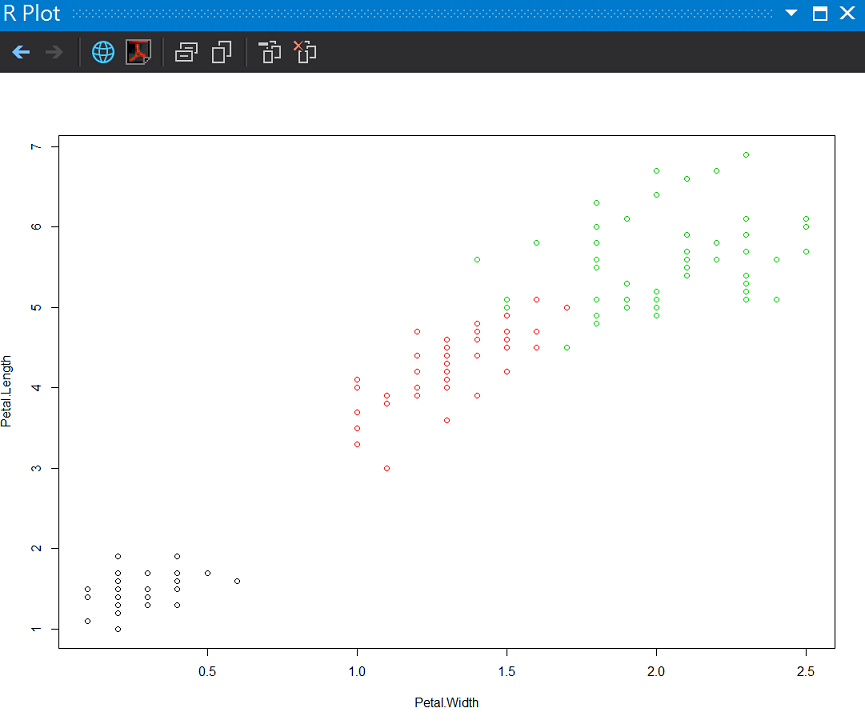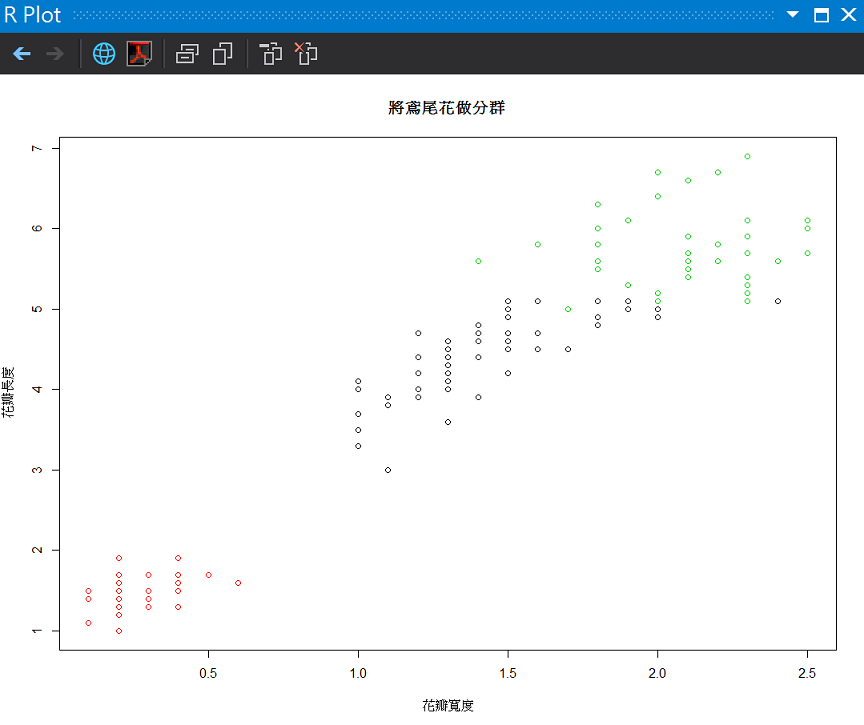``````ggplot(data, aes(x = Petal.Length, y = Petal.Width)) +
geom_point(aes(color = factor(km\$cluster))) +
stat_density2d(aes(color = factor(km\$cluster)))
``````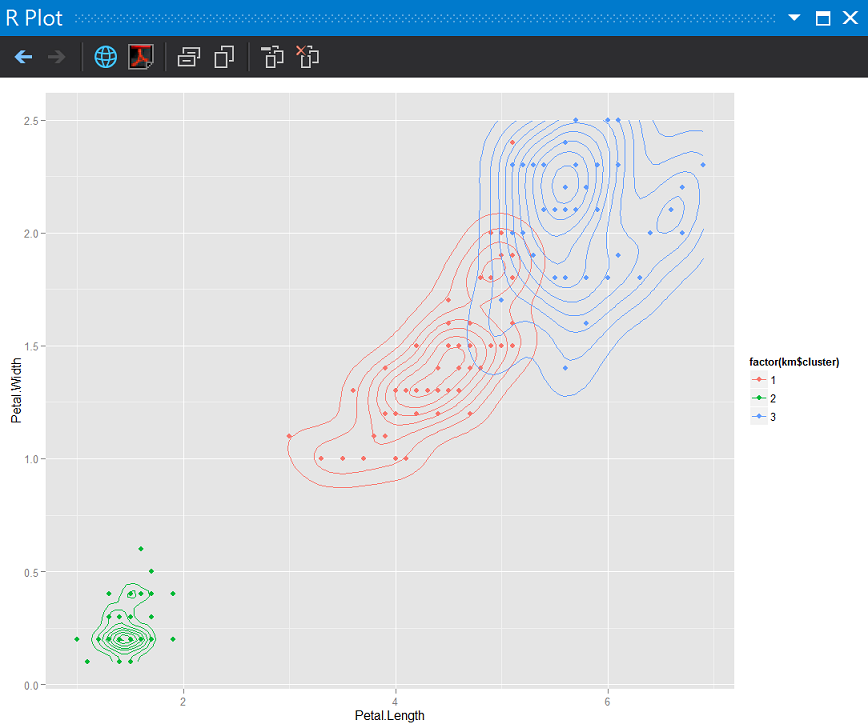## 評估分群模型

• 組內距離平方和WSS(Within Cluster Sum of Squares) 越小越好
• 組間距離平方和BSS(Between Cluster Sum of Squares) 越大越好
• 總離均差平方和TSS(Total Cluster Sum of Squares)
``````(WSS <- km\$tot.withinss)
(BSS <- km\$betweenss)
(TSS <- BSS + WSS)
(ratio <- WSS / TSS)
``````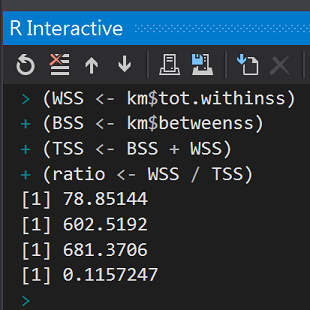## K值的決定

``````library(ggplot2)
klist <- seq(1:10)
knnFunction <- function(x) {
kms <- kmeans(data, centers = x, nstart = 1)
ratio <- kms\$tot.withinss / (kms\$tot.withinss + kms\$betweenss)
}
ratios <- sapply(klist, knnFunction)

# k value與準確度視覺化
df <- data.frame(
kv = klist, KMratio = accuracies)

ggplot(df, aes(x = kv, y = KMratio, label = kv, color = KMratio)) +
geom_point(size = 5) + geom_text(vjust = 2)
``````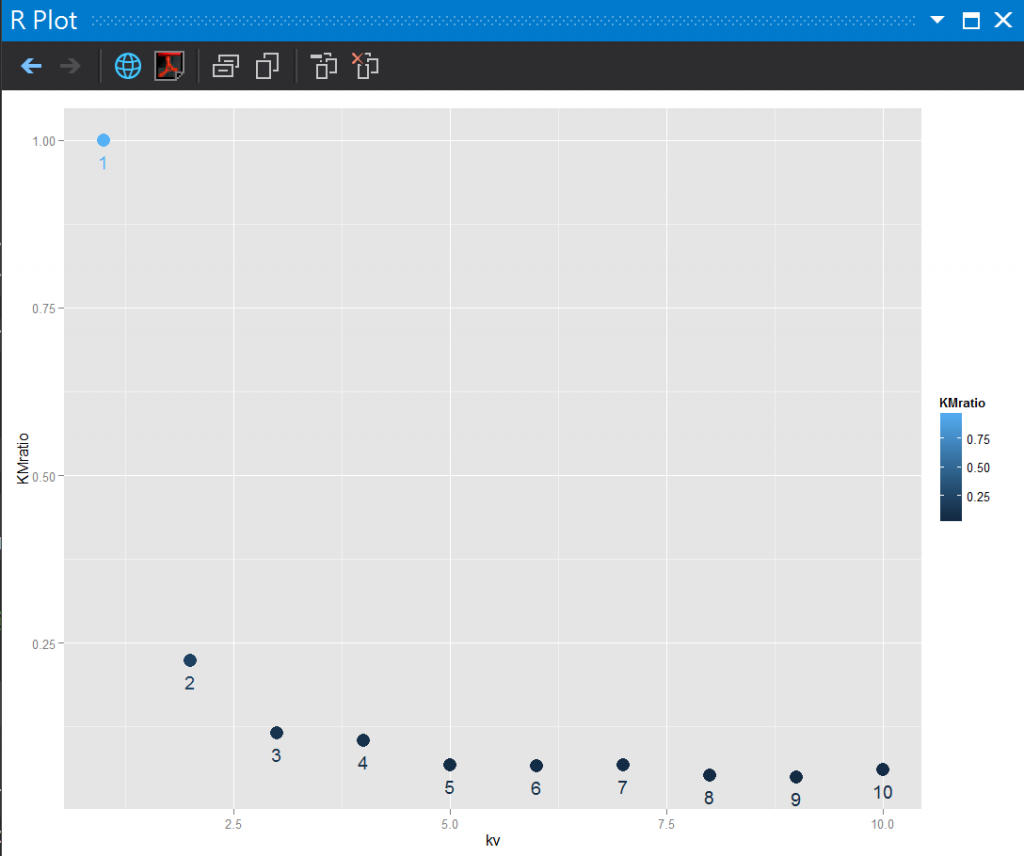• 取出k值最小但WSS/TSS越貼近0的k value。
• 那個1-mean好像有分等於沒分，WSS/TSS ratio值為1。
• 最後就是雖然都有k，但k平均演算法與k近鄰演算法沒有任何關係。

## 參考2013.05攝於vienna,Austria

R語言與機器學習見面會30